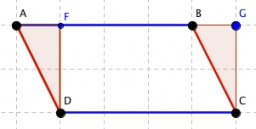# Parallelogram 25371

A parallelogram with a side length of 5 cm and a height to this side length of 4 cm has the same area as an isosceles triangle with a base length of 5 cm. What is the height of this triangle?

h2 =  8 cm

### Step-by-step explanation:Did you find an error or inaccuracy? Feel free to write us. Thank you!

Tips for related online calculators
Need help calculating sum, simplifying, or multiplying fractions? Try our fraction calculator.
Do you have a linear equation or system of equations and looking for its solution? Or do you have a quadratic equation?
Calculation of an isosceles triangle.